# Overview

$${\tt BigVAR}$$ is the companion R package to the papers “VARX-L: Structured Regularization for Large Vector Autoregression with Exogenous Variables” (Joint with David Matteson and Jacob Bien) and “High Dimensional Forecasting via Interpretable Vector Autoregression (HLag)” (Joint with Ines Wilms, David Matteson, and Jacob Bien).

$${\tt BigVAR}$$ allows for the simultaneous estimation and forecasting of high-dimensional time series by applying structured penalties to the standard vector autoregression (VAR) and vector autoregression with exogenous variables (VARX) frameworks. This is useful in many applications which make use of time-dependent data, such as macroeconomics, finance, and internet traffic, as the conventional VAR and VARX are heavily overparameterized. In addition, as stated in Ghysels and Marcellino (2018), VARs with “large enough” lag order can adequately approximate VARMA models.

Our package adapts solution methods from the regularization literature to a multivariate time series setting, allowing for the computationally efficient estimation of high-dimensional VAR and VARX models.

We also allow for least squares refitting based on the nonzero support selected by our procedures as well as the ability to incorporate mild non-stationarity by shrinking toward a vector random walk. For more information on these extensions, we refer you to our papers Nicholson, Matteson, and Bien (2017b) and Nicholson et al. (2020).

This vignette presents a brief formal overview of our notation, the models contained in $${\tt BigVAR}$$, and the functionality of the package. For an interactive tutorial see the shiny app. Any questions or feature requests with regard to $${\tt BigVAR}$$ can be addressed to . If you have basic questions about VAR or multivariate time series in general, we recommend consulting Lütkepohl (2005).

Notation and Methodology provides an overview of the VARX model as well as the $${\tt BigVAR}$$ framework. Our penalty structures are described in VARX-L and HLAG. Empirical penalty parameter selection procedures are discussed in Penalty Parameter Selection and N-fold cross validation. Package specific syntax is detailed in BigVAR Details. Finally, example applications and extensions of $${\tt BigVAR}$$ are provided in Selecting a Structure and Impulse Response Functions.

## Installation

The stable version of $${\tt BigVAR}$$ is available on cran. The developmental release can be installed from github using the following command:

install_github("wbnicholson/BigVAR/BigVAR")

## Quick Start

In this section, we provide a basic overview of the capabilities of $${\tt BigVAR}$$. Further sections will provide elaboration as to the full functionality of $${\tt BigVAR}$$.

$$\mathbf{Y}$$, a simulated multivariate time series of dimension $$100\times 3$$ is included with $${\tt BigVAR}$$ and is used throughout this vignette (details as to its construction are provided in Example Data). It can be accessed by calling:

library(BigVAR)
data(Y)

In order to forecast $$\hat{y}_{t+1}$$ using a vector autoregression with a lasso penalty $$\lambda=1$$ and maximum lag order of 2, one can simply run

# 3 x 7 coefficient matrix
B = BigVAR.fit(Y,struct='Basic',p=2,lambda=1)[,,1]
# construct 7 x 99 lag matrix of Y
#>   .. ..$trainZ: num [1:12, 1:96] -0.0529 0.1385 0.0501 0.0174 -0.4301 ... #> ..@ lagmax : num 4 #> ..@ Structure : chr "Basic" #> ..@ Relaxed : logi FALSE #> ..@ Granularity : num [1:2] 150 10 #> ..@ intercept : logi TRUE #> ..@ Minnesota : logi FALSE #> ..@ horizon : num 1 #> ..@ verbose : logi FALSE #> ..@ crossval : chr "Rolling" #> ..@ ic : logi TRUE #> ..@ VARX : list() #> ..@ T1 : num 33 #> ..@ T2 : num 66 #> ..@ ONESE : logi FALSE #> ..@ ownlambdas : logi FALSE #> ..@ tf : logi FALSE #> ..@ alpha : num 0.25 #> ..@ recursive : logi FALSE #> ..@ dates : chr(0) #> ..@ constvec : num [1:3] 1 1 1 #> ..@ tol : num 1e-04 #> ..@ window.size : num 0 #> ..@ separate_lambdas : logi FALSE #> ..@ loss : chr "L2" #> ..@ delta : num 2.5 #> ..@ gamma : num 3 #> ..@ rolling_oos : logi FALSE #> ..@ linear : logi FALSE #> ..@ refit_fraction : num 1 $${\tt BigVAR.results}$$ also has a plot method, to show a comparison of in-sample MSFE over the grid of $$\lambda$$ values. plot(results)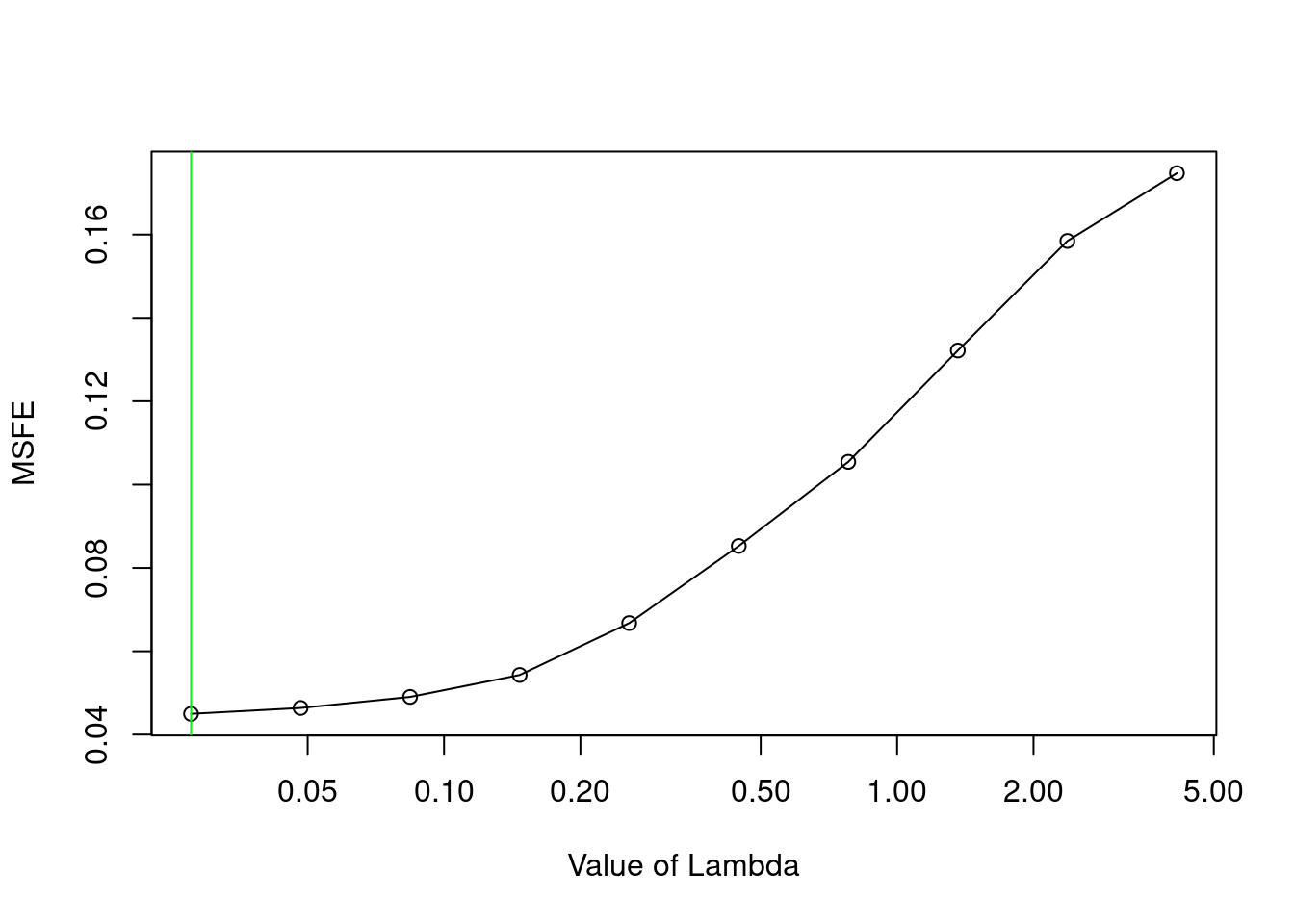Generally, you want this graph to have a parabolic shape with the optimal value in one of the middle indices. In this scenario, since the slope of the line is very flat, it is likely that increasing the depth of the grid (i.e. the first parameter of $${\tt gran}$$ in $$\tt{constructModel}$$) would not substantially improve forecasts. It is not recommended to make the depth too large as it substantially increases computation time.  mod2<-constructModel(Y,p=4,"Basic",gran=c(5,10),h=1,cv="Rolling",verbose=FALSE,IC=FALSE) res2=cv.BigVAR(mod2) plot(res2)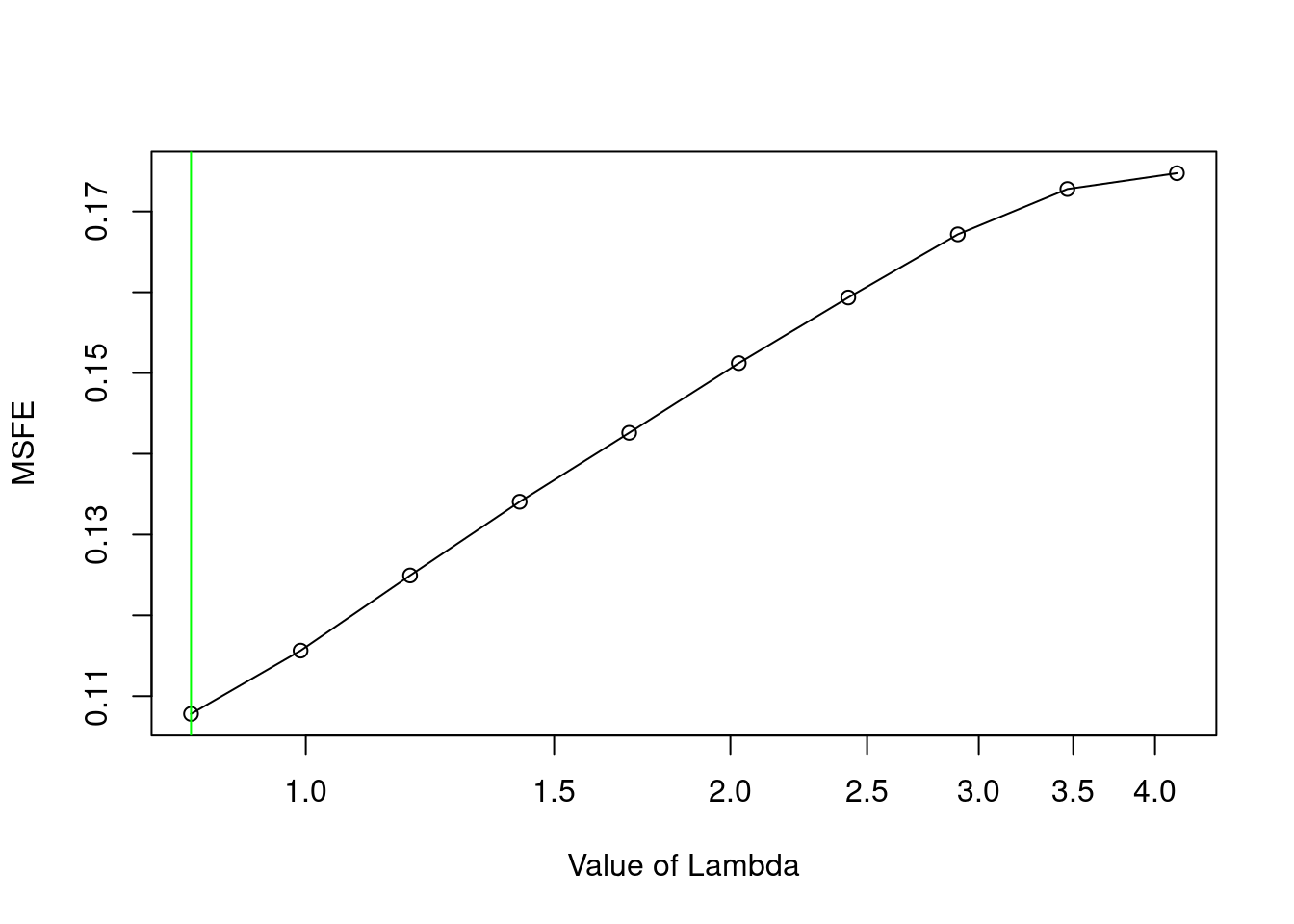However, since the slope of the line in this case is quite steep, it is likely that forecasts will be improved by increasing the depth.  mod3<-constructModel(Y,p=4,"Basic",gran=c(500,10),h=1,cv="Rolling",verbose=FALSE,IC=FALSE) res3=cv.BigVAR(mod3) plot(res3)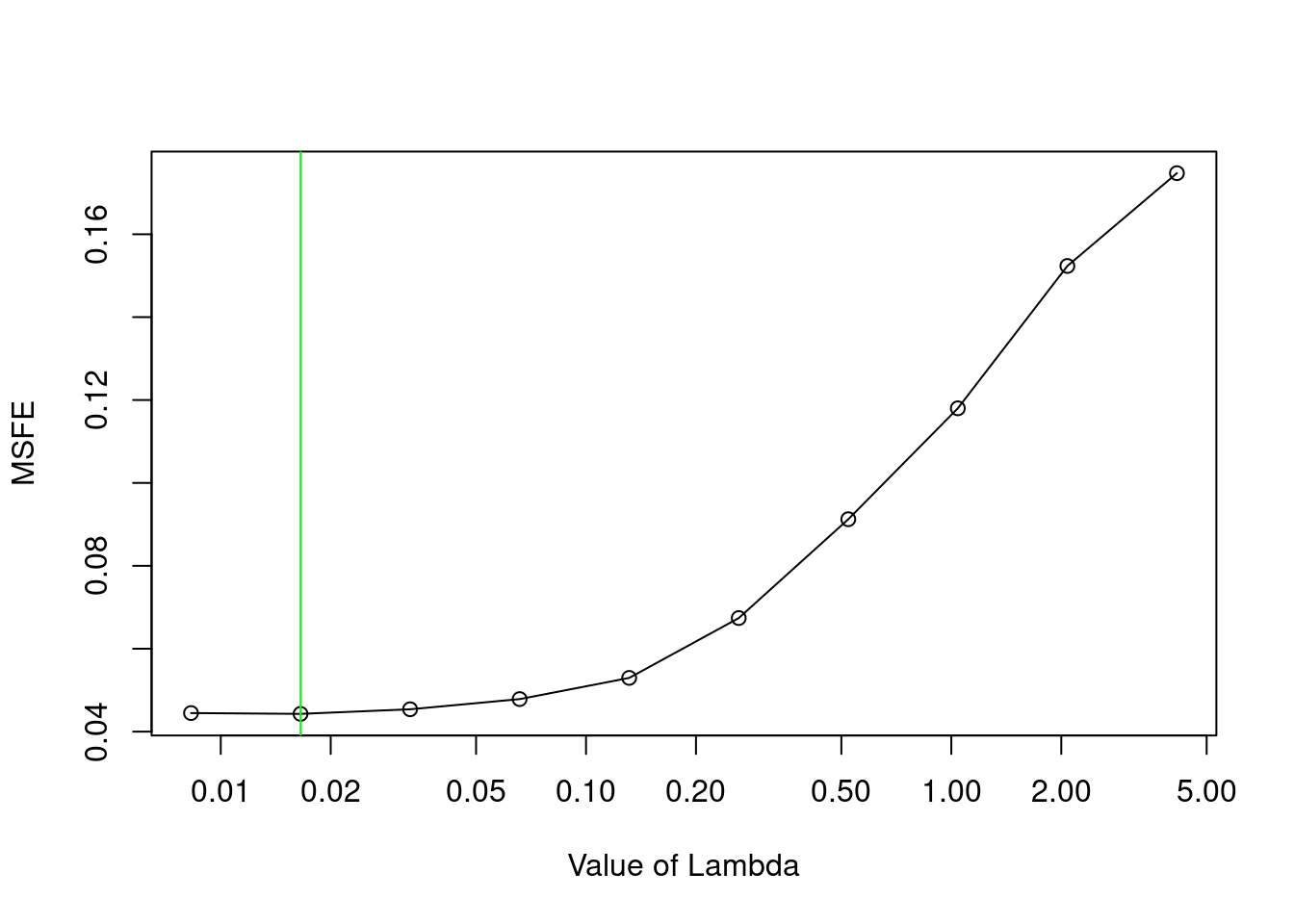As evidenced above, this plot does not always take on a parabolic shape. On occasion, when the grid is very deep, it will start to level off. In this scenario, it is best to decrease the depth of the grid. ## Prediction We can also view the sparsity pattern of the final estimated coefficient matrix with $${\tt \verb|SparsityPlot.BigVAR.results|}$$ SparsityPlot.BigVAR.results(results)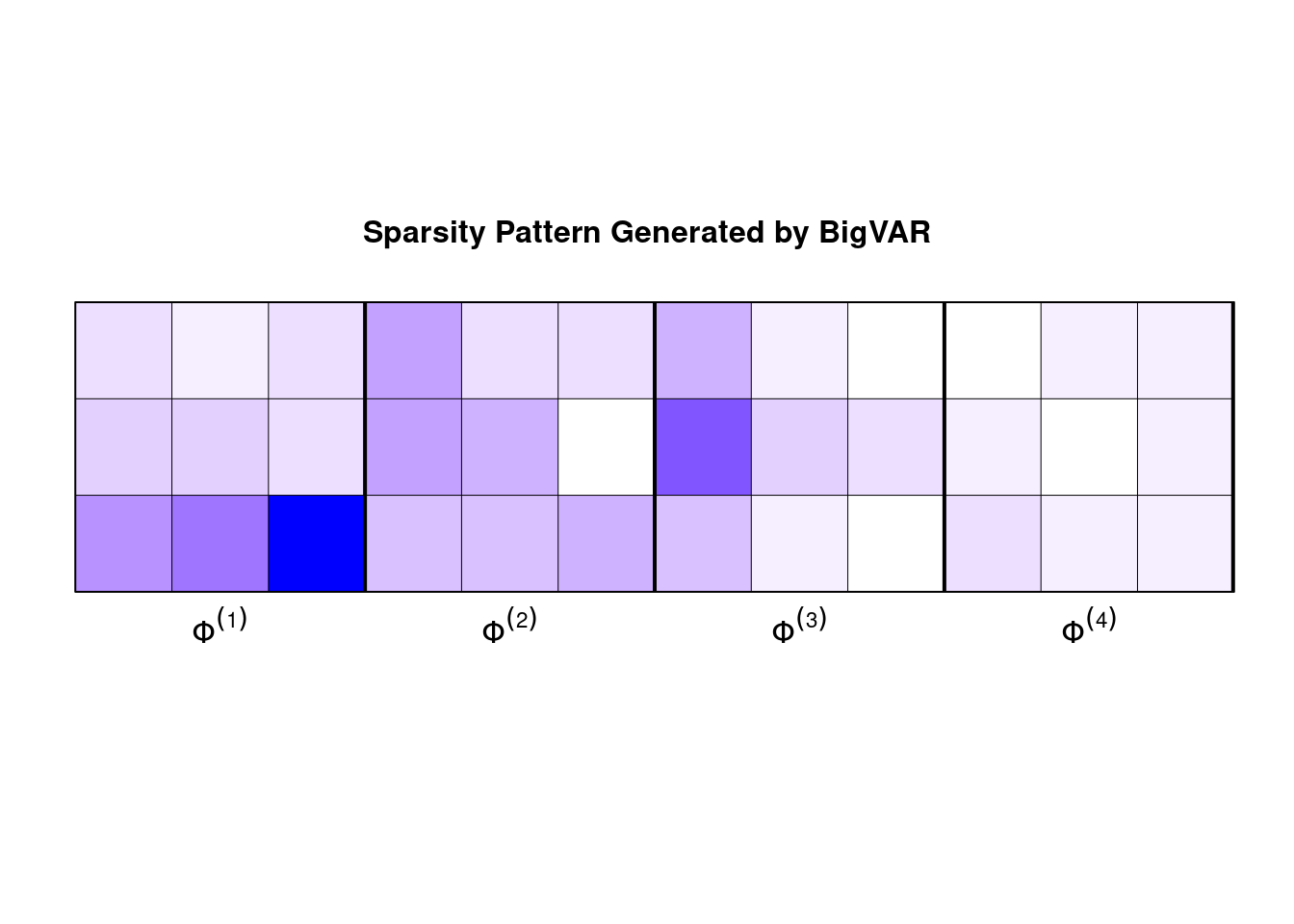Finally, out of sample predictions can be computed with $${\tt predict}$$ predict(results,n.ahead=1) #> [,1] #> [1,] 0.1031721 #> [2,] 0.2602539 #> [3,] 0.1656290 95 percent confidence intervals can be returned with the option $${\tt confint=TRUE}$$ predict(results,n.ahead=1, confint=TRUE) #> forecast lower upper #> 1 0.1031721 0.08491428 0.1214299 #> 2 0.2602539 0.24132051 0.2791873 #> 3 0.1656290 0.14474386 0.1865141 ## Coefficients A formatted dataframe of the coefficient matrix of the final iteration of forecast evaluation can be obtained via the $${\tt coef}$$ method coef(results) #> intercept Y1L1 Y2L1 Y3L1 Y1L2 Y2L2 #> Y1 0.00618473 -0.1727210 -0.04200019 0.10349734 -0.4860618 -0.09321464 #> Y2 0.01118086 -0.2468233 -0.20468901 0.09407572 -0.4877787 -0.39656465 #> Y3 -0.01605536 -0.5412728 0.77566736 1.26037684 0.3483038 -0.30552614 #> Y3L2 Y1L3 Y2L3 Y3L3 Y1L4 Y2L4 #> Y1 -0.1249840 -0.4504043 0.01020169 0.00000000 0.00000000 -0.03681427 #> Y2 0.0000000 -0.9731988 -0.23008984 -0.09409432 0.08253306 0.00000000 #> Y3 -0.3755885 0.3463590 0.01076152 0.00000000 0.16266747 -0.02062425 #> Y3L4 #> Y1 0.07901527 #> Y2 0.06713842 #> Y3 -0.02940889 ## Example Data $${\tt Y}$$, the sparse multivariate time series included with $${\tt BigVAR}$$ was generated using matrix $$\mathbf{A}$$, included with $${\tt BigVAR}$$ as $${\tt Generator}$$. The sparsity structure of $$\mathbf{A}$$ is visualized in the following plot data(Y) # simulated multivariate time series # coefficient matrix used to generate Y data(Generator) # note that coefficients with a darker shade are larger in magnitude SparsityPlot(A[1:3,],p=4,3,s=0,m=0,title="Sparsity Structure of Generator Matrix")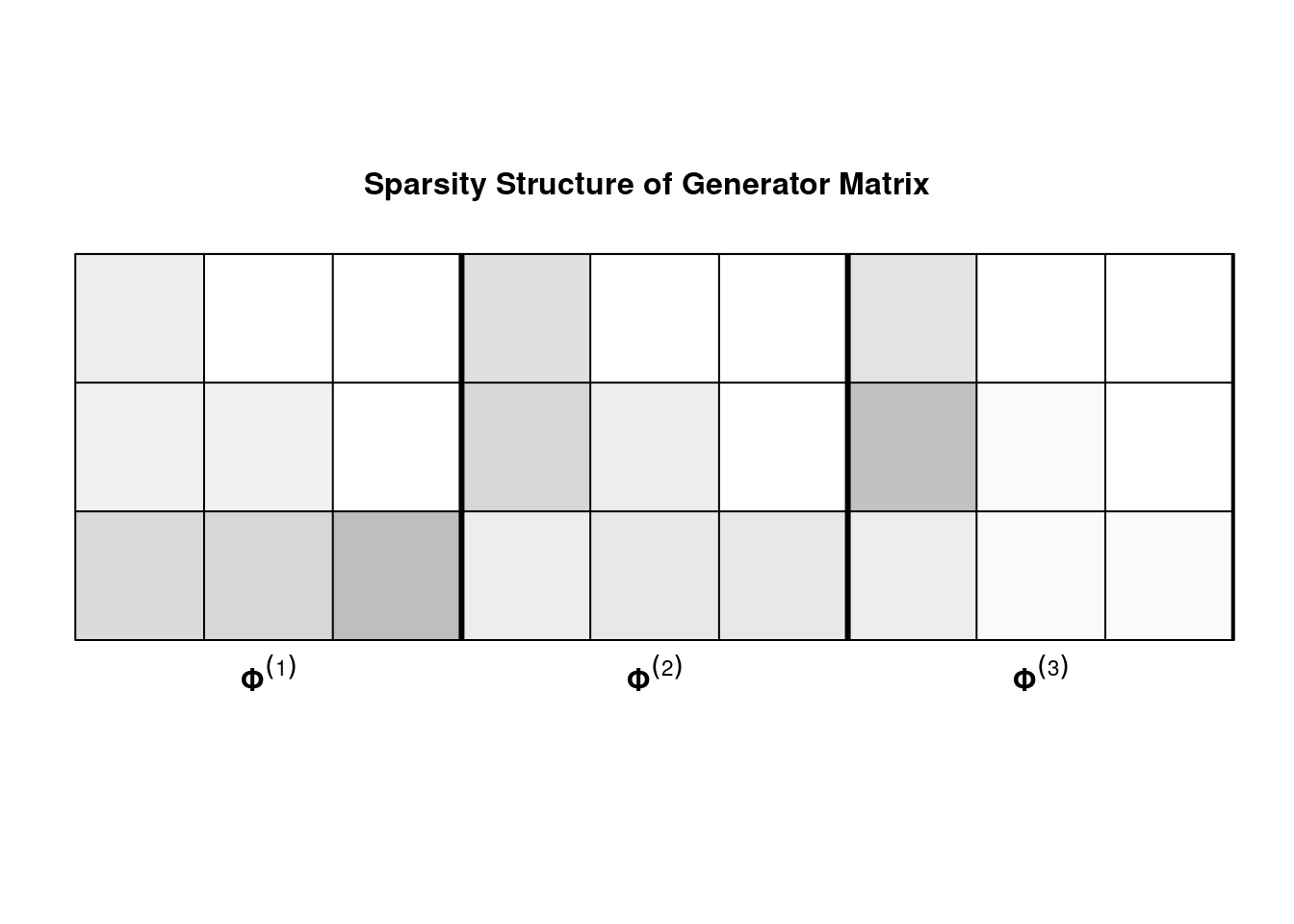In order to generate multivariate VARs, we transform $$k\times kp$$ coefficient matrix to its multiple companion form (i.e. converting to a $$kp\times kp$$ matrix representing a VAR of lag order 1). For details, consult page 15 of Lütkepohl (2005). # Extensions ## Fit with fixed, known $$\lambda$$ In certain scenarios, it may be overly cumbersome to construct a $$\tt{BigVAR}$$ object and perform rolling validation or lambda grid construction (for example, out-of-sample testing once an “optimal” penalty parameter has been selected). As an alternative, we include the function $$\tt{\verb|BigVAR.fit|}$$ which will fit a $${\tt BigVAR}$$ model with a fixed penalty parameter without requiring the construction of a $${\tt BigVAR}$$ object. # fit a Basic VARX-L with k=2,m=1,s=2,p=4,lambda=.01 VARX=list(k=2,s=2) #returns k x (kp+ms+1) coefficient matrix model=BigVAR.fit(Y,p,"Basic",lambda=1e-2,VARX=VARX,intercept=TRUE) model #> , , 1 #> #> [,1] [,2] [,3] [,4] [,5] [,6] #> [1,] 0.001528357 -0.1720469 -0.09112866 -0.5342244 -0.0492386 -0.4740068 #> [2,] 0.013302701 -0.2715414 -0.22482794 -0.5224687 -0.4526648 -1.0502028 #> [,7] [,8] [,9] [,10] [,11] [,12] #> [1,] 0.05114002 0.0000000 -0.08945957 -0.007923539 0.1239634 0.08961456 #> [2,] -0.23898735 0.0488135 -0.04731603 -0.085209092 0.0000000 0.10005671 #> [,13] #> [1,] -0.06588215 #> [2,] -0.02683559 ## N-fold cross validation If, instead of rolling or “leave one out” validation, you wish to use a custom procedure to set the penalty parameters, you can do so using repeated calls to $${\tt \verb|BigVAR.fit|}$$. As an example, we provide a N-fold cross validation function (which does not respect time dependence).  # N-fold cross validation for VAR # Y: data # nfolds: number of cross validation folds # struct: penalty structure # p: lag order # nlambdas: number of lambdas: # gran1: depth of lambda grid # seed: set to make it reproducible NFoldcv <- function(Y,nfolds,struct,p,nlambdas,gran1,seed) { MSFE <- matrix(0,nrow=nrow(Y),ncol=10) A <- constructModel(Y,p,struct=struct,gran=c(gran1,nlambdas),verbose=F) # construct lag matrix Z1 <- VARXLagCons(Y,X=NULL,s=0,p=p,0,0) trainZ <- Z1$Z[2:nrow(Z1$Z),] trainY <- matrix(Y[(p+1):nrow(Y),],ncol=ncol(Y)) set.seed(seed) inds <- sample(nrow(trainY)) B <- BigVAR.est(A) lambda.grid <- B$lambda
folds <- cut(inds,breaks=nfolds,labels=FALSE)

MSFE <- matrix(0,nrow=nfolds,ncol=nlambdas)
for(i in 1:nfolds){

test <- trainY[which(folds==i),]
train <- trainY[which(folds!=i),]
testZ <-t(t(trainZ)[which(folds!=i),])

B=BigVAR.fit(train,p=p,lambda=lambda.grid,struct='Basic')

#iterate over lambdas
for(j in 1:nlambdas){

MSFETemp <- c()
for(k in 1:nrow(test))    {
tempZ <- testZ[,k,drop=FALSE]
bhat <- matrix(B[,2:dim(B),j],nrow=ncol(Y),ncol=(p*ncol(Y)))
preds <- B[,1,j]+bhat%*%tempZ

MSFETemp <- c(MSFETemp,sum(abs(test[k,]-preds))^2)

}
MSFE[i,j] <- mean(MSFETemp)

}

}

return(list(MSFE=MSFE,lambdas=lambda.grid))
}
# 10 fold cv
MSFEs<-NFoldcv(Y,nfolds=10,"Basic",p=5,nlambdas=10,gran1=50,seed=2000)
# choose smaller lambda in case of ties (prevents extremely sparse solutions)
opt=MSFEs$lambda[max(which(colMeans(MSFEs$MSFE)==min(colMeans(MSFEs$MSFE))))] opt #>  5.60583 ## Information Criterion Benchmarks We have noticed a relative dearth of packages that allow for the estimation and forecasting of VAR and VARX models via least squares with lag order selected according to an information criterion. By default, $$\tt{cv.BigVAR}$$ returns least squares AIC and BIC benchmarks as forecast comparison. $$\tt{VARXFit}$$ will fit a VAR or VARX model with pre-specified maximum lag orders and the function $$\tt{VARXForecastEval}$$ will evaluate the forecasting performance of VAR and VARX models with information criterion selected by AIC or BIC over a user-specified time horizon. The arguments to $$\tt{VARXForecastEval}$$ are detailed below: 1. $${\tt Y}$$: $$T\times k$$ matrix of endogenous (modeled) series. 2. $${\tt X}$$: $$T\times m$$ matrix of exogenous (unmodeled) series. 3. $${\tt p}$$: Maximum lag order for endogenous series. 4. $${\tt s}$$: Maximum lag order for exogenous series. 5. $${\tt T1}$$: Integer, Start of forecast evaluation period. 6. $${\tt T2}$$: Interger, End of forecast evaluation period. 7. $${\tt IC}$$: Information criterion used (“AIC” or “BIC”) 8. $${\tt h}$$: Forecast horizon. 9. $${\tt Iterated}$$: Logical, indicator to use “iterated” (default $$\tt{FALSE}$$ indicating that direct forecasts are used). Note that one may encounter scenarios in which the number of least squares VARX parameters $$(k^2\times p+m*k*s) > (k+m)T$$. Our algorithm will terminate lag order selection as soon as the problem becomes ill-posed. In the event that the problem is ill-conditioned at $$p=1$$, the algorithm will always return a lag order of zero. An example usage of $$\tt{VARXForecastEval}$$ is below. data(Y) p <- 4 T1 <- floor(nrow(Y))/3 T2 <- floor(2*nrow(Y))/3 #Matrix of zeros for X X <- matrix(0,nrow=nrow(Y),ncol=ncol(Y)) BICMSFE <- VARXForecastEval(Y,X,p,0,T1,T2,"BIC",1) In addition, one-step ahead predictions from VARX models can be computed using the function $$\tt{PredictVARX}$$. mod <- VARXFit(Y,3,NULL,NULL) pred <-PredictVARX(mod) pred #>  0.1017921 0.2531906 0.1622109 ## Selecting a Structure The choice of structured penalty is not always clear at the outset of the forecasting problem. Since our methods are all computationally manageable across most dimensions, one approach that we recommend is to use a subset of the data to fit models with all applicable structured penalties and find the set of “superior models” using the $$\tt{MCSProcedure}$$ function from the package $$\tt{MCS}$$. For more information about the package and procedure consult Bernardi and Catania (2018) and the original paper Hansen, Lunde, and Nason (2011). We will start by simulating a $$\text{VAR}_3(6)$$ library(MASS) k=3;p=6 B=matrix(0,nrow=k,ncol=p*k) A1<- matrix(c(.4,-.07,.08,-.06,-.7,.07,-.08,.07,-.4),ncol=3,nrow=3) A2 <- matrix(c(-.6,0,0,0,-.4,0,0,0,.5),ncol=3,nrow=3) B[,1:k]=A1 B[,(5*k+1):(6*k)]=A2 A <- VarptoVar1MC(B,p,k) set.seed(2000) Y <-MultVarSim(k,A,p,.005*diag(k),500) SparsityPlot(B,p,k,0,0, title='Sparsity Plot of VAR Coefficient Matrix')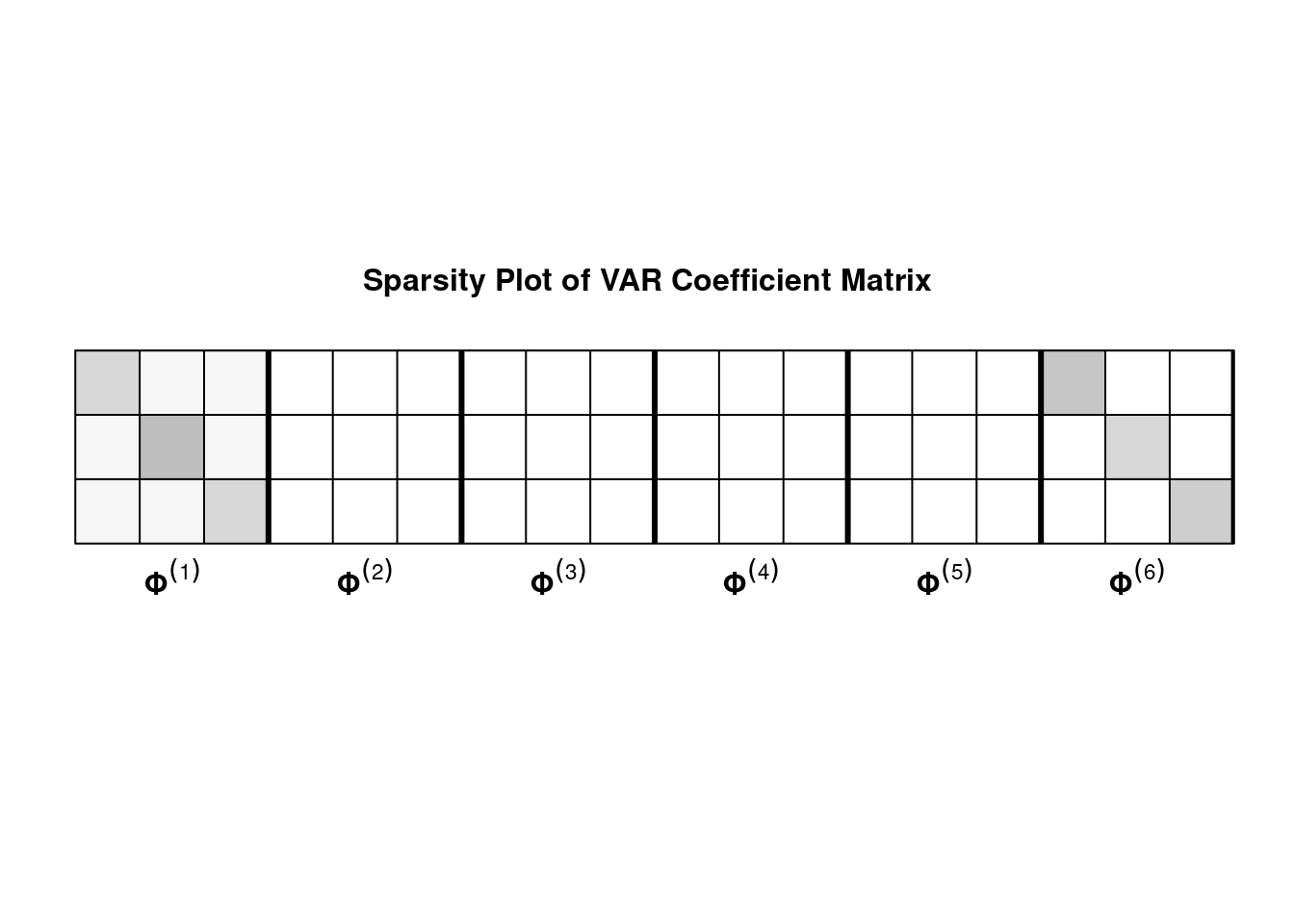The first lag matrix and the own lags in the fifth coefficient matrix are the only nonzero entries. This suggests that the structures incorporating an Own/Other or Lag type penalty will achieve the best forecast performance. There is no within-group sparsity so we should not expect the Sparse counterparts to be in the set of superior models. library(MCS) # train on first 250 observations YTrain=Y[1:250,] Loss <- c() T1=1*floor(nrow(YTrain)/3);T2=2*floor(nrow(YTrain)/3) p=8 structures<-c("Basic","BasicEN","Lag","SparseLag","OwnOther","HLAGC","HLAGOO","HLAGELEM","MCP","SCAD") for(i in structures){ # construct BigVAR object; we will perform a dual grid search for the sparse lag and sparse own/other models if(i%in%c("SparseLag","SparseOO")){ alpha=seq(0,1,length=10) }else{ alpha=0 } A<- constructModel(YTrain,p=p,struct=i,gran=c(100,10),T1=T1,T2=T2,verbose=FALSE,model.controls=list(intercept=FALSE,alpha=alpha)) # perform rolling cv res<- cv.BigVAR(A) # save out of sample loss for each structure Loss <- cbind(Loss,res@OOSMSFE) } # construct AIC and BIC benchmarks BIC <- VARXForecastEval(YTrain,matrix(0,nrow=nrow(YTrain)),p,0,T2,nrow(YTrain),"BIC",1)$MSFE
AIC <- VARXForecastEval(YTrain,matrix(0,nrow=nrow(YTrain)),p,0,T2,nrow(YTrain),"AIC",1)\$MSFE

Loss <- as.data.frame(Loss)
names(Loss) <- structures
Loss <- cbind(Loss,BIC,AIC)

names(Loss)[(ncol(Loss)-1):ncol(Loss)] <- c("BIC","AIC")
names(Loss) <- paste0(names(Loss),"L")
mcs.test <- MCSprocedure(as.matrix(Loss),verbose=FALSE)
mcs.test
#>
#> ------------------------------------------
#> -          Superior Set of Models        -
#> ------------------------------------------
#>           Rank_M       v_M  MCS_M Rank_R       v_R  MCS_R       Loss
#> LagL           2  1.511579 0.1336      2  1.511579 0.1336 0.01397682
#> OwnOtherL      1 -1.511579 1.0000      1 -1.511579 1.0000 0.01376844
#>
#> Details
#> ------------------------------------------
#>
#> Number of eliminated models  :   10
#> Statistic    :   Tmax
#> Elapsed Time :   Time difference of 17.68301 secs

As expected, we find that the set of superior models contains only the Own/Other VAR-L and Lag VAR-L.

## Impulse Response Functions

(Note: this section is adapted from the Nicholson, Matteson, and Bien (2017a).)

Though $${\tt BigVAR}$$ is primarily designed to forecast high-dimensional time series, it can also be of use in analyzing the joint dynamics of a group of interrelated time series. In order to conduct policy analysis, many macroeconomists make use of VARs to examine the impact of shocks to certain variables on the entire system (holding all other variables fixed). This is know as impulse response analysis.

For example, a macroeconomist may wish to analyze the impact of a 100 basis point increase in the Federal Funds Rate on all included series over the next 8 quarters. To do so, we can utilize the function $${\tt generateIRF}$$, which converts the last estimated $${\tt BigVAR}$$ coefficient matrix to fundamental form.

We use the following function to generate an impulse response function:

suppressMessages(library(expm))

# Phi k x kp coefficient matrix
# sigma kxk residual covariance matrix
# n number of time steps to run IRF
# p lag order
# k number of series
# Y0: k dimensional vector reflecting initialization of the IRF
generateIRF <- function(Phi,Sigma,n,k,p,Y0)
{

if(p>1){

A <-VarptoVar1MC(Phi,p,k)

}else{

A <- Phi

}
J <- matrix(0,nrow=k,ncol=k*p)
diag(J) <- 1
P <- t(chol(Sigma))
IRF <- matrix(0,nrow=k,ncol=n+1)
for(i in 0:n)
{

phi1 <- J%*%(A%^%i)%*%t(J)

theta20 <- phi1%*%P

IRF[,i+1] <- (theta20%*%Y0)

}

return(IRF)

}
require(quantmod)
#>
#> Attaching package: 'zoo'
#> The following objects are masked from 'package:base':
#>
#>     as.Date, as.Date.numeric
#> Registered S3 method overwritten by 'quantmod':
#>   method            from
#>   as.zoo.data.frame zoo
require(zoo)
# get GDP, Federal Funds Rate, CPI from FRED
#Gross Domestic Product (Relative to 2000)
getSymbols('GDP',src='FRED',type='xts')
#> 'getSymbols' currently uses auto.assign=TRUE by default, but will
#> use auto.assign=FALSE in 0.5-0. You will still be able to use
#> and getOption("getSymbols.auto.assign") will still be checked for
#> alternate defaults.
#>
#> This message is shown once per session and may be disabled by setting
#> options("getSymbols.warning4.0"=FALSE). See ?getSymbols for details.
#>  "GDP"
GDP<- aggregate(GDP,as.yearqtr,mean)
GDP <- GDP/mean(GDP["2000"])*100
# Transformation Code: First Difference of Logged Variables
GDP <- diff(log(GDP))
index(GDP) <- as.yearqtr(index(GDP))
# Federal Funds Rate
getSymbols('FEDFUNDS',src='FRED',type='xts')
#>  "FEDFUNDS"
FFR <- aggregate(FEDFUNDS,as.yearqtr,mean)
# Transformation Code: First Difference
FFR <- diff(FFR)
# CPI ALL URBAN CONSUMERS, relative to 1983
getSymbols('CPIAUCSL',src='FRED',type='xts')
#>  "CPIAUCSL"
CPI <- aggregate(CPIAUCSL,as.yearqtr,mean)
CPI <- CPI/mean(CPI['1983'])*100
# Transformation code: difference of logged variables
CPI <- diff(log(CPI))
getSymbols('M1SL',src='FRED',type='xts')
#>  "M1SL"
M1<- aggregate(M1SL,as.yearqtr,mean)
# Transformation code, difference of logged variables
M1 <- diff(log(M1))
# combine series
Y <- cbind(CPI,FFR,GDP,M1)
names(Y) <- c("CPI","FFR","GDP","M1")
Y <- na.omit(Y)
k=ncol(Y)
T <- nrow(Y)
# start/end of rolling validation
T1 <- which(index(Y)=="1985 Q1")
T2 <- which(index(Y)=="2005 Q1")

#Demean
Y <- Y - (c(rep(1, nrow(Y))))%*%t(c(apply(Y[1:T1,], 2, mean)))
#Standarize Variance
for (i in 1:k) {
Y[, i] <- Y[, i]/apply(Y[1:T1,], 2, sd)[i]
}
library(expm)
# Fit an Elementwise HLAG model
Model1=constructModel(as.matrix(Y),p=4,struct="HLAGELEM",gran=c(25,10),verbose=FALSE,VARX=list(),T1=T1,T2=T2)
Model1Results=cv.BigVAR(Model1)

# generate IRF for 10 quarters following a 1 percent increase in the federal funds rate
IRFS <- generateIRF(Phi=Model1Results@betaPred[,2:ncol(Model1Results@betaPred)],Sigma=cov(Model1Results@resids),n=10,k=ncol(Y),p=4,Y0=c(0,.01,0,0))

IRFS <- generateIRF(Model1Results@betaPred[,2:ncol(Model1Results@betaPred)],cov(Model1Results@resids),10,4,4,c(0,.01,0,0))    

The impulse responses generated from this “shock” are depicted below.